Next: Caveat concerning the blind Up: Approximate methods Previous: Evaluation of type B   Contents

## Examples of type B uncertainties

1. Previous measurements of other particular quantities, performed in similar conditions, have provided a repeatability standard deviation6.4 of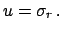: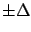2. A manufacturer's calibration certificate states that the uncertainty, defined asstandard deviations, is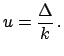'':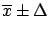3. A result is reported in a publication as, stating that the average has been performed on four measurements and the uncertainty is a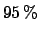confidence interval. One has to conclude that the confidence interval has been calculated using the Student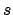: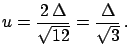4. A manufacturer's specification states that the error on a quantity should not exceed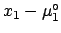. With this limited information one has to assume a uniform distribution: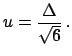5. A physical parameter of a Monte Carlo is believed to lie in the interval ofaround its best value, but not with uniform distribution: the degree of belief that the parameter is at centre is higher than the degree of belief that it is at the edges of the interval. With this information a triangular distribution can be reasonably assumed: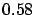Note that the coefficient in front ofchanges from the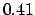of the previous example to the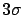of this. If the intervalwere a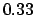interval then the coefficient would have been equal to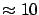. These variations -- to be considered extreme -- are smaller than the statistical fluctuations of empirical standard deviations estimated from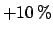measurements. This shows that one should not be worried that the type B uncertainties are less accurate than type A, especially if one tries to model the distribution of the physical quantity honestly.
6. The absolute energy calibration of an electromagnetic calorimeter module is not known exactly and is estimated to be between the nominal one and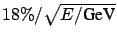. The statistical'' error is known by test beam measurements to be. What is the uncertainty on the energy measurement of an electron which has apparently released 30 GeV?
• There is no type A uncertainty, since only one measurement has been performed.
• The energy has to be corrected for the best estimate of the calibration constant: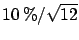, with an uncertainty of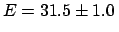due to sampling (the statistical'' error):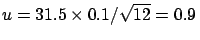GeV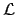• Then one has to take into account the uncertainty due to absolute energy scale calibration:
• assuming a uniform distribution of the true calibration constant,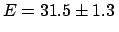GeV: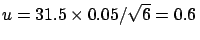GeV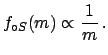• assuming, more reasonably, a triangular distribution,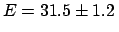GeV,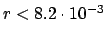GeV• Interpreting the maximum deviation from the nominal calibration as uncertainty (see comment at the end of Section),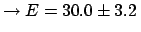GeV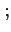GeV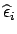As already mentioned earlier in these notes, while reasonable assumptions (in this case the first two) give consistent results, this is not true if one makes inconsistent use of the information just for the sake of giving safe'' uncertainties.
7. Note added: the original version of the primer contained at this point a more realistic and slightly more complicated example'', which requires, instead, a next-to-linear treatment , which was not included in the notes, neither is it in this new version. Therefore, I prefer to skip this example in order to avoid confusion.Next: Caveat concerning the blind Up: Approximate methods Previous: Evaluation of type B   Contents
Giulio D'Agostini 2003-05-15